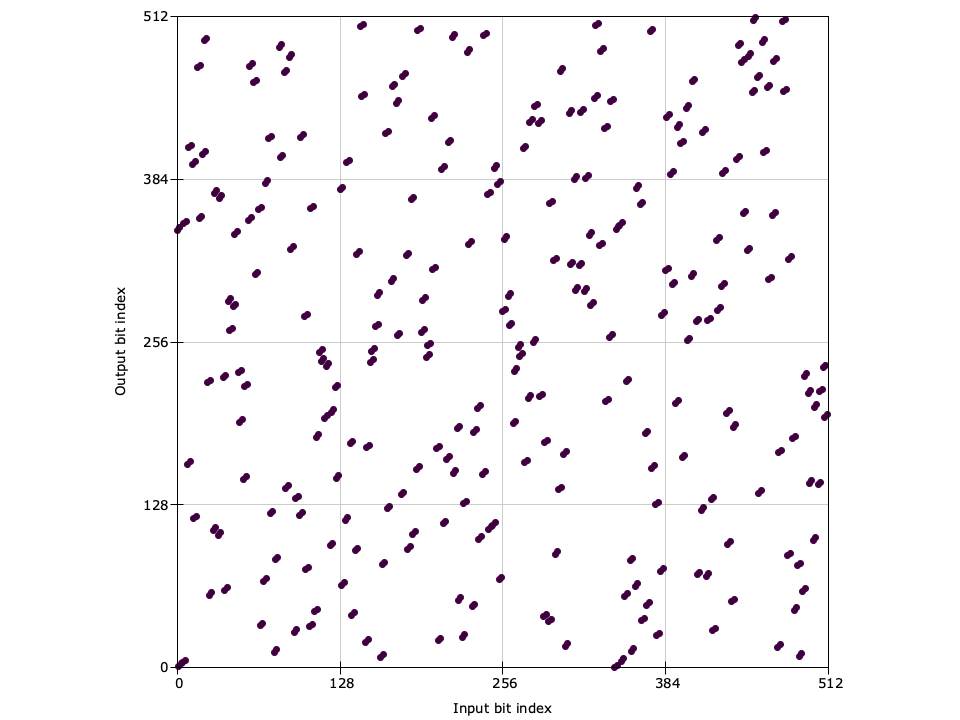# Interleaver

This section describes the functionality of the liquid interleaver object. In wireless communications systems, bit errors are often grouped together as a result of multi-path fading, demodulator symbol errors, and synchronizer instability. Interleavers serve to distribute grouped bit errors evenly throughout a block of data which aids certain forward error-correction (FEC) codes in their decoding process (see [section-fec] on error-correcting codes). On the transmit side of the wireless link, the interleaver re-orders the bits after FEC encoding and before modulation. On the receiving side, the de-interleaver re-shuffles the bits to their original position before attempting to run the FEC decoder. The bit-shuffling order must be known at both the transmitter and receiver.

## Description of Operation

The interleaver object operates by permuting indices on the input data sequence. The indices are computed during the interleaver_create() method and stored internally. At each iteration data bytes are re-shuffled using the permutation array. Depending upon the properties of the array, multiple iterations should not result in observing the original data sequence. Shown below is a simple example where 8 symbols ( $$0,\ldots,7$$ ) are re-ordered using a random permutation. The data at iteration 0 are the original data which are permuted twice.


forward
permutation     iter     iter     iter
0 -> 6          0           6           1
1 -> 4          1           4           3
2 -> 7          2           7           5
3 -> 0          3           0           6
4 -> 3          4           3           0
5 -> 2          5           2           7
6 -> 1          6           1           4
7 -> 5          7           5           2


Reversing the process is as simple as computing the reverse permutation from the input; this is equivalent to reversing the arrows in the forward permutation (e.g. the $$2 \rightarrow 7$$ forward permutation becomes the $$7 \rightarrow 2$$ reverse permutation).


reverse
permutation     iter     iter     iter
0 -> 3          1           6           0
1 -> 6          3           4           1
2 -> 5          5           7           2
3 -> 4          6           0           3
4 -> 1          0           3           4
5 -> 7          7           2           5
6 -> 0          4           1           6
7 -> 2          2           5           7


Notice that permuting indices only re-orders the bytes of data and does nothing to shuffle the bits within the byte. It is beneficial to FEC decoders to separate the bit errors as much as possible. Therefore, in addition to index permutation, liquid also applies masks to the data while permuting.

## Interface

The interleaver object operates like most objects in liquid with typical create() , destroy() , and execute() methods.

• interleaver_create(n) creates an interleaver object accepting $$n$$ bytes, and defaulting to 2 iterations.
• interleaver_destroy(q) destroys the interleaver object, freeing all internally-allocated memory arrays.
• interleaver_set_num_iterations(q,k) sets the number of iterations of the interleaver. Increasing the number of iterations helps improve bit dispersion, but can also increase execution time. The default number of iterations at the time of creation is 2 (see [fig-framing-interleaver-scatterplot] ).
• interleaver_encode(q,*msg_dec,*msg_enc) runs the forward interleaver, reading data from the first array argument and writing the result to the second array argument. The array pointers can reference the same block of memory, if necessary.
• interleaver_decode(q,*msg_enc,*msg_dec) runs the reverse interleaver, reading data from the first array argument and writing the result to the second array argument. Like the encode() method, the array pointers can reference the same block of memory.

This listing gives a basic demonstration to the interface to the interleaver object:


#include <liquid/liquid.h>

int main() {
// options
unsigned int n=9; // message length (bytes)

// create the interleaver
interleaver q = interleaver_create(n);
interleaver_set_depth(q,3);

// create arrays
unsigned char msg_org[n];   // original message data
unsigned char msg_int[n];   // interleaved data
unsigned char msg_rec[n];   // de-interleaved, recovered data

// ...initialize msg_org...

// interleave/de-interleave the data
interleaver_encode(q, msg_org, msg_int);
interleaver_decode(q, msg_int, msg_rec);

// destroy the interleaver object
interleaver_destroy(q);
}


Figure [fig-framing-interleaver-scatterplot]. interleaver (block) demonstration of a 64-byte (512-bit) array with increasing number of iterations (interleaving depth)}Figure [fig-interleaver-scatterplot-0]. Iteration 0Figure [fig-interleaver-scatterplot-1]. Iteration 1Figure [fig-interleaver-scatterplot2]. Iteration 2Figure [fig-interleaver-scatterplot3]. Iteration 3Figure [fig-interleaver-scatterplot-4]. Iteration 4

A visualization of the interleaver can be seen in [fig-framing-interleaver-scatterplot] where the input index is plotted against the output index for varying number of iterations. Notice that with zero iterations, the output and input are identical (no interleaving). With one iteration only the bytes are interleaved, and so the output is grouped into 8-bit blocks. Further iterations, however, result in sufficiently dispersed bits, and patterns between input and output indices become less evident. The packetizer object ( [section-framing-packetizer] ) uses the interleaver object in conjunction to forward error-correction coding ( [section-fec] ) to provide a simple interface for generating protected data packets. A full example can be found in examples/interleaver_example.c .